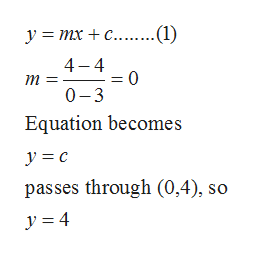# Write an equation a) in slope intercept form and b) in standard form for the line passing through (3, 4) and perpendicular to X equals negative 7

Question
22 views

Write an equation a) in slope intercept form and b) in standard form for the line passing through (3, 4) and perpendicular to X equals negative 7

check_circle

Step 1

Given that line is passing through the point (3, 4) and perpendicular to x = -7.

Step 2

The equation of line perpendicular to x = -7 is given by y = b. Since this line is passing through (3, 4) so,

Step 3

Since (3, 4) and (0, 4) are the points on th...help_outlineImage Transcriptionclosey = Mx + c........(1) 4 4 = 0 0-3 т Equation becomes y c passes through (0,4), so y =4 fullscreen

### Want to see the full answer?

See Solution

#### Want to see this answer and more?

Solutions are written by subject experts who are available 24/7. Questions are typically answered within 1 hour.*

See Solution
*Response times may vary by subject and question.
Tagged in

### Other